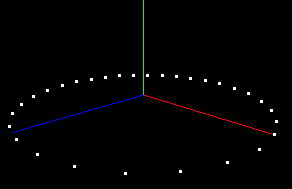# Python Tutorial

## Python API

The python API is designed for ease of use. For speed critical applications, C++ API should be used. Looping in python is extremely slow. It is hoped that in the future a mapping to numpy might be provided to allow manipulating particles in a SIMD fashion. Nevertheless, python API remains useful for manipulating particles.

To use Partio's python API first import partio as

``` import partio
```

Help on functions that are available are shown in

``` help(partio)
```

## Creating a Particle Set

To create a particle set and add a couple of attributes one could write

```particleSet=partio.create()
P=particleSet.addAttribute("position",partio.VECTOR,3)
V=particleSet.addAttribute("velocity",partio.VECTOR,3)
id=particleSet.addAttribute("id",partio.INT,1)
```

Once this is done, we could add a series of particles that form a circle

```n=30
radiansPer=2*math.pi/n
particleSet.addParticles(n)
for i in range(n):
particleSet.set(P,i,(math.cos(i*radiansPer),0,math.sin(i*radiansPer)))
particleSet.set(V,i,(0,0,0))
particleSet.set(id,i,(i,))
```

Finally, we can write the particle file into a BGEO by writing

```partio.write("circle.bgeo",particleSet) # write uncompressed
partio.write("circle.bgeo",particleSet,True) # write compressed
partio.write("circle.bgeo.gz",particleSet) # write compressed
```

We can then visualize the particle set with

```partview circle.bgeo
```

yielding the image## Loading a particle set

Loading a particle set is relatively easy. If you only want to know how many particles are available or what headers are available you can do

```>>> pHeaders=partio.readHeaders("circle.bgeo")
```

If you want everything associated with the file

```>>> p=partio.read("circle.bgeo")
```

{document}

## Finding nearest neighbors

A KD-Tree mode is supported in partio. To use it, you must first sort the particles into a KD-Tree. This is done with the |sort()| function. Once that is done a query can be done. The basic query requires a maximum distance to look for particles as well as a maximum number of particles to return. For example, we could read our circle back in and look for particles nearby (1,0,0) like so:

```p=partio.read("circle.bgeo")
p.sort()
p.findNPoints((1.,0.,0.),.1,3)
```

Generated on 5 Apr 2011 for Partio by1.6.1